# Practice Paper cutting Questions and Answers

## Paper Folding & Paper Cutting

The topic of Paper folding & Paper cutting is a part of Non-Verbal reasoning and forms an important part of the Reasoning syllabus of many government and competitive exams like- SSC CGL, SSC CPO, SBI PO, IBPS PO, IBPS SO and many others.

### What is Paper Folding and Paper Cutting?

• Paper folding is a process in which a piece of paper (with a certain embedded design pattern) is folded along a certain dotted line and the candidates are required to select the correct folded pattern.
• Questions based on paper folding consist of a question figure representing a transparent sheet and four answer figures. The transparent sheet contains some figural patterns and one/more dotted lines. These dotted lines indicate the axis along which the paper is folded.

Let us look at an example  to understand-

Q1) Directions: In each of the following problems, a square transparent sheet with a pattern is given. Figure out from amongst the four alternatives as to how the pattern would appear when the transparent sheet is folded along the dotted line.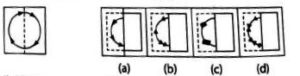Solution: (c)

Explanation:

The right half of the transparent sheet is being folded along the dotted line and placed on the left half of the sheet. Thus, the figure obtained resembles the answer figure (c).

• On the other hand, paper cutting is a process by which a piece of paper (of any shape) is folded along a certain direction and then cuts are made into it.
• In questions based on paper cutting, one or more question figures are given showing the manner in which a piece of paper is folded, where a dotted line is the reference line along which the paper has to be folded and the arrow indicates the direction of the fold. Then the paper is punched (cut) from a particular section and such cuts may be based on varying patterns. These patterns appear on the paper when the paper is unfolded after cutting.

Let us look at an example-

Q1) Directions: In the following question, a piece of paper is folded and cut as shown below in the question figures. From the given answer figures, indicate how it will appear when opened?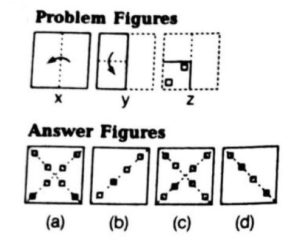Solution: (a)

Explanation:

In these types of questions, the easiest thing to do is to start with the last picture and undo the steps taken in the question. Let us see, if we start from the figure z, and move to y, we will get a figure that has square cuts as half diagonals in the left half only. From the figure y we have to now move to the figure x, that would give a figure similar to the one in (a). Hence the correct answer here is (a).

### Sample Questions on Paper Folding and Paper Cutting

Q1) A square transparent sheet with a pattern is given. Figure out from among the four alternatives as to how the pattern would appear when the transparent sheet is folded at the middle line shown?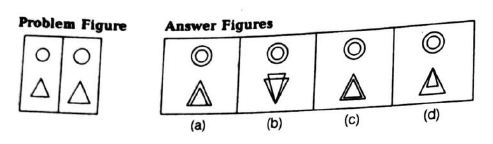• A
• B
• C
• D

Solution: (D)

Explanation:

If we look at the options, we would see that the option (a) can’t be the answer because if the sheet was folded at the middle the triangles will be joined at the vertex. Similarly, we can discard all the other options except (d). The correct option is (d).

Q2) A piece of paper is folded and punched as shown below in the question figures. from the given answer figures, indicate how it will appear when opened?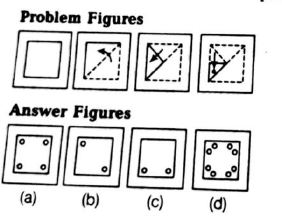• A
• B
• C
• D

Solution: (D)

Explanation:

We see that the paper is folded in the form of a right triangle and the cut is made near the midpoint of the hypotenuse inside the triangle. Unfolding the triangle would give us another right triangle which has marks near the two sides apart from the hypotenuse. At this point, we can say that the answer could be either (a) or (d). Similarly, if we keep unfolding we will see that the correct answer is (d).

Q3) A piece of paper is folded and punched as shown below in the question figures. From the given answer figures, indicate how it will appear when opened?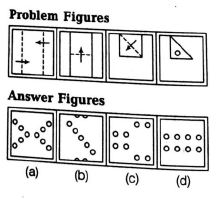• A
• B
• C
• D

Solution: (A)

Explanation:

We will begin with the last problem figure and move on to the first one, while tracing out all the spots on each step. When we do that we will see that out of the four options, the only one that represents the answer figure is the one in option (a).

Q4) A triangular sheet of paper has been folded and punched as shown in the following series of figures. How will it appear when opened?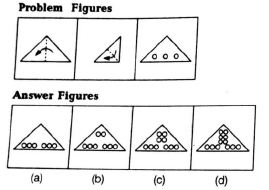• A
• B
• C
• D

Solution: (D)

Q5) A square piece of paper cut at the edges as shown is taken and folded as shown below. How would the folded pattern look?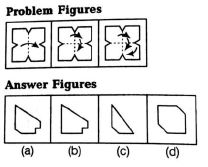• A
• B
• C
• D

Solution: (B)

Q6) Find out from amongst the four alternatives as to how the pattern would appear when the transparent sheet is folded at the dotted line.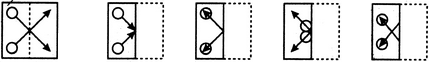(X)                     (A)                 (B)                 (C)                 (D)

• A
• B
• C
• D

Solution: (B)

Q7) Choose a figure which would most closely resemble the unfolded form of Figure (Z).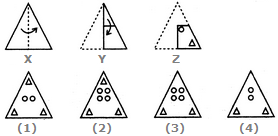• 1
• 2
• 3
• 4

Solution: (c )

Q8) Choose a figure which would most closely resemble the unfolded form of Figure (Z).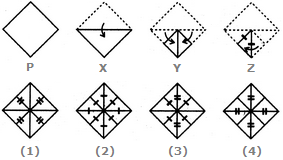• 1
• 2
• 3
• 4

Solution: (b)

Q9) Find out from amongst the four alternatives as to how the pattern would appear when the transparent sheet is folded at the dotted line.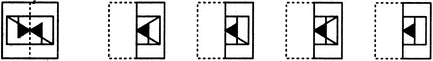(X)                     (1)                 (2)                 (3)                 (4)

• 1
• 2
• 3
• 4

Solution: (c )

Q10) Find out from amongst the four alternatives as to how the pattern would appear when the transparent sheet is folded at the dotted line.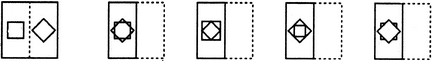(X)                     (1)                 (2)                 (3)                 (4)

• 1
• 2
• 3
• 4

Solution: (a)

### Conclusion

Paper Cutting & Paper Folding is a tricky topic as it involves visualization of the question and answer figures. However, candidates can master this topic by practicing a different variety of questions. Candidates can expect 2 to 3 questions in competitive exams.

×
×